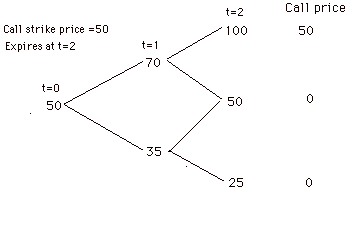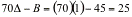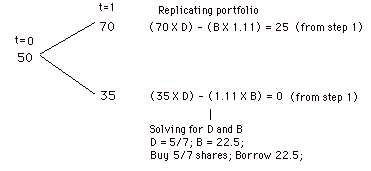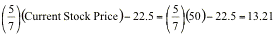## Options Arbitrage

As derivative securities, options differ from futures in a very important respect. They represent rights rather than obligations – calls gives you the right to buy and puts gives you the right to sell. Consequently, a key feature of options is that the losses on an option position are limited to what you paid for the option, if you are a buyer. Since there is usually an underlying asset that is traded, you can, as with futures, construct positions that essentially are riskfree by combining options with the underlying asset.

### Exercise Arbitrage

The easiest arbitrage opportunities in the option market exist when options violate simple pricing bounds. No option, for instance, should sell for less than its exercise value. With a call option: Value of call > Value of Underlying Asset – Strike Price

With a put option: Value of put > Strike Price – Value of Underlying Asset

For instance, a call option with a strike price of \$ 30 on a stock that is currently trading at \$ 40 should never sell for less than \$ 10. It it did, you could make an immediate profit by buying the call for less than \$ 10 and exercising right away to make \$ 10.

In fact, you can tighten these bounds for call options, if you are willing to create a portfolio of the underlying asset and the option and hold it through the option’s expiration.  The bounds then become:

With a call option: Value of call > Value of Underlying Asset – Present value of Strike Price

With a put option: Value of put > Present value of Strike Price – Value of Underlying Asset

Too see why, consider the call option in the previous example. Assume that you have one year to expiration and that the riskless interest rate is 10%.

Present value of Strike Price = \$ 30/1.10 = \$27.27

Lower Bound on call value = \$ 40 - \$27.27 = \$12.73

The call has to trade for more than \$12.73. What would happen if it traded for less, say \$ 12? You would buy the call for \$ 12, sell short a share of stock for \$ 40 and invest the net proceeds of \$ 28 (\$40 – 12) at the riskless rate of 10%. Consider what happens a year from now:

If the stock price > 30: You first collect the proceeds from the riskless investment (\$28(1.10) =\$30.80), exercise the option (buy the share at \$ 30) and cover your short sale. You will then get to keep the difference of \$0.80.

If the stock price < 30: You collect the proceeds from the riskless investment (\$30.80), but a share in the open market for the prevailing price then (which is less than \$30) and keep the difference.

In other words, you invest nothing today and are guaranteed a positive payoff in the future. You could construct a similar example with puts.

The arbitrage bounds work best for non-dividend paying stocks and for options that can be exercised only at expiration (European options). Most options in the real world can be exercised only at expiration (American options) and are on stocks that pay dividends. Even with these options, though, you should not see short term options trading violating these bounds by large margins, partly because exercise is so rare even with listed American options and dividends tend to be small. As options become long term and dividends become larger and more uncertain, you may very well find options that violate these pricing bounds, but you may not be able to profit off them.

### Replicating Portfolio

One of the key insights that Fischer Black and Myron Scholes had about options in the 1970s that revolutionized option pricing was that a portfolio composed of the underlying asset and the riskless asset could be constructed to have exactly the same cash flows as a call or put option. This portfolio is called the replicating portfolio. In fact, Black and Scholes used the arbitrage argument to derive their option pricing model by noting that since the replicating portfolio and the traded option had the same cash flows, they would have to sell at the same price.

To understand how replication works, let us consider a very simple model for stock prices where prices can jump to one of two points in each time period. This model, which is called a binomial model, allows us to model the replicating portfolio fairly easily. In the figure below, we have the binomial distribution of a stock, currently trading at \$ 50 for the next two time periods. Note that in two time periods, this stock can be trading for as much as \$ 100 or as little as \$ 25. Assume that the objective is to value a call with a strike price of 50, which is expected to expire in two time periods:Now assume that the interest rate is 11%. In addition, define

= Number of shares in the replicating portfolio

B = Dollars of borrowing in replicating portfolio

The objective is to combine shares of stock and B dollars of borrowing to replicate the cash flows from the call with a strike price of 50. Since we know the cashflows on the option with certainty at expiration, it is best to start with the last period and work back through the binomial tree.

Step 1: Start with the end nodes and work backwards. Note that the call option expires at t=2, and the gross payoff on the option will be the difference between the stock price and the exercise price, if the stock price > exercise price, and zero, if the stock price < exercise price.The objective is to construct a portfolio of D shares of stock and B in borrowing at t=1,  when the stock price is \$ 70, that will have the same cashflows at t=2 as the call option with a strike price of 50.  Consider what the portfolio will generate in cash flows under each of the two stock price scenarios, after you pay back the borrowing with interest (11% per period) and set the cash flows equal to the cash flows you would have received on the call.

If stock price = \$ 100:             Portfolio Value = 100 D – 1.11 B = 50

If stock price = \$ 50:               Portfolio Value =   50 D – 1.11 B =   0

Drawing on skills that most of us have not used since high school, we can solve for both the number of shares of stock you will need to buy (1) and the amount you will need to borrow (\$ 45) at t=1.  Thus, if the stock price is \$70 at t=1, borrowing \$45 and buying one share of the stock will give the same cash flows as buying the call. To prevent arbitrage, the value of the call at t=1, if the stock price is \$70, has to be equal to the cost (to you as an investor) of setting up the replicating position:

Value of Call = Cost of Replicating Position =Considering the other leg of the binomial tree at t=1,If the stock price is 35 at t=1, then the call is worth nothing.

Step 2: Now that we know how much the call will be worth at t=1 (\$25 if the stock price goes to \$ 70 and \$0 if it goes down to \$ 35), we can move backwards to the earlier time period and create a replicating portfolio that will provide the values that the option will provide.In other words, borrowing \$22.5 and buying 5/7 of a share wtoday ill provide the same cash flows as a call with a strike price of \$50. The value of the call therefore has to be the same as the cost of creating this position.

Value of Call = Cost of replicating position =Consider for the moment the possibilities for arbitrage if the call traded at less than \$13.21, say \$ 13.00. You would buy the call for \$13.00 and sell the replicating portfolio for \$13.21 and claim the difference of \$0.21. Since the cashflows on the two positions are identical, you would be exposed to no risk and make a certain profit. If the call trade for more than \$13.21, say \$13.50, you would buy the replicating portfolio, sell the call and claim the \$0.29 difference. Again, you would not have been exposed to any risk.

You could construct a similar example using puts. The replicating portfolio in that case would be created by selling short on the underlying stock and lending the money at the riskless rate. Again, if puts are priced at a value different from the replicating portfolio, you could capture the difference and be exposed to no risk.

What are the assumptions that underlie this arbitrage? The first is that both the traded asset and the option are traded and that you can trade simultaneously in both markets, thus locking in your profits. The second is that there are no (or at least very low transactions costs). If transactions costs are large, prices will have to move outside the band created by these costs for arbitrage to be feasible. The third is that you can borrow at the riskless rate and sell short, if necessary. If you cannot, arbitrage may no longer be feasible.

### Arbitrage across options

When you have multiple options listed on the same asset, you may be able to take advantage of relative mispricing – how one option is priced relative to another - and lock in riskless profits.  We will look first at the pricing of calls relative to puts and then consider how options with different exercise prices and maturities should be priced, relative to each other.

#### Put-Call Parity

When you have a put and a call option with the same exercise price and the same maturity, you can create a riskless position by selling the call, buying the put and buying the underlying asset at the same time. To see why, consider selling a call and buying a put with exercise price K and expiration date t, and simultaneously buying the underlying asset at the current price S. The payoff from this position is riskless and always yields K at expiration t. To see this, assume that the stock price at expiration is S*. The payoff on each of the positions in the portfolio can be written as follows:

 Position Payoffs at t if S*>K Payoffs at t if S*

Since this position yields K with certainty, the cost of creating this position must be equal to the present value of K at the riskless rate (K e-rt).

S+P-C = K e-rt

C - P = S - K e-rt

This relationship between put and call prices is called put call parity. If it is violated, you have arbitrage.

If C-P > S – Ke-rt, you would sell the call, buy the put and buy the stock. You would earn more than the riskless rate on a riskless investment.

If C-P < S – Ke-rt, you would buy the call, sell the put and sell short the stock. You would then invest the proceeds at the riskless rate and end up with a riskless profit at maturity.

Note that put call parity creates arbitrage only for options that can be exercised only at maturity (European options) and may not hold if options can be exercise early (American options).

Does put-call parity hold up in practice or are there arbitrage opportunities? One study examined option pricing data from the Chicago Board of Options from 1977 to 1978 and found potential arbitrage opportunities in a few cases. However, the arbitrage opportunities were small and persisted only for short periods. Furthermore, the options examined were American options, where arbitrage may not be feasible even if put-call parity is violated.  A more recent study by Kamara and Miller of options on the S&P 500 (which are European options) between 1986 and 1989 finds fewer violations of put-call parity.

#### Mispricing across Strike Prices and Maturities

A spread is a combination of two or more options of the same type (call or put) on the same underlying asset. You can combine two options with the same maturity but different exercise prices (bull and bear spreads), two options with the same strike price but different maturities (calendar spreads), two options with different exercise prices and maturities (diagonal spreads) and more than two options (butterfly spreads). You may be able to use spreads to take advantage of relative mispricing of options on the same underlying stock.

Strike Prices: A call with a lower strike price should never sell for less than a call with a higher strike price, assuming that they both have the same maturity. If it did, you could buy the lower strike price call and sell the higher strike price call, and lock in a riskless profit. Similarly, a put with a lower strike price should never sell for more than a put with a higher strike price and the same maturity. If it did, you could buy the higher strike price put, sell the lower strike price put and make an arbitrage profit.

Maturity: A call (put) with a shorter time to expiration should never sell for more than a call (put) with the same strike price with a long time to expiration. If it did, you would buy the call (put) with the shorter maturity and sell (put) the call with the longer maturity (i.e, create a calendar spread) and lock in a profit today. When the first call expires, you will either exercise the second call (and have no cashflows) or sell it (and make a further profit).

Even a casual perusal of the option prices listed in the newspaper each day should make it clear that it is very unlikely that pricing violations that are this egregious will exist in a market as liquid as the Chicago Board of Options.